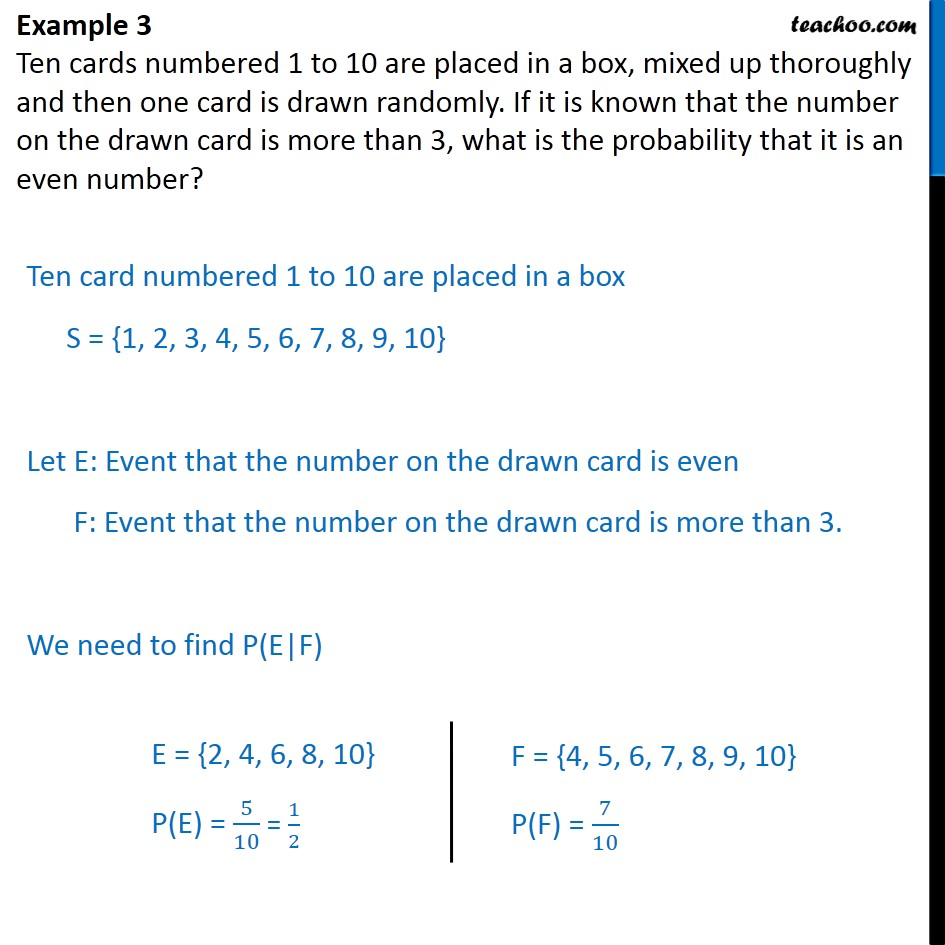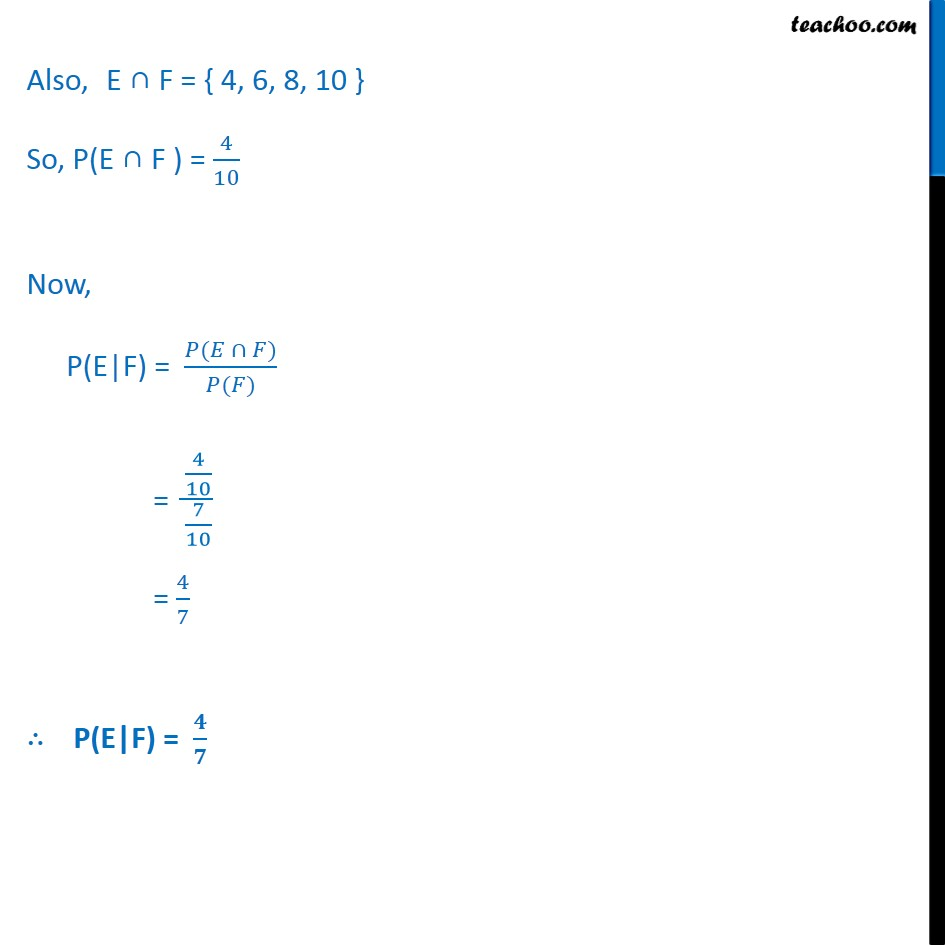Examples

Chapter 13 Class 12 Probability
Serial order wiseLearn in your speed, with individual attention - Teachoo Maths 1-on-1 Class

### Transcript

Example 3 Ten cards numbered 1 to 10 are placed in a box, mixed up thoroughly and then one card is drawn randomly. If it is known that the number on the drawn card is more than 3, what is the probability that it is an even number? Ten card numbered 1 to 10 are placed in a box S = {1, 2, 3, 4, 5, 6, 7, 8, 9, 10} Let E: Event that the number on the drawn card is even F: Event that the number on the drawn card is more than 3. We need to find P(E|F) Also, E ∩ F = { 4, 6, 8, 10 } So, P(E ∩ F ) = 4﷮10﷯ Now, P(E|F) = 𝑃(𝐸 ∩ 𝐹)﷮𝑃(𝐹)﷯ = 4﷮10﷯﷮ 7﷮10﷯﷯ = 4﷮7﷯ ∴ P(E|F) = 𝟒﷮𝟕﷯